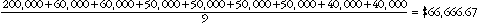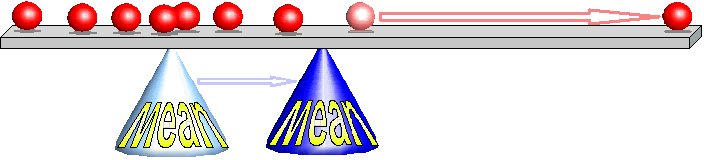1332.0.55.002 - Statistical Language!, 2008
Latest ISSUE Released at 11:30 AM (CANBERRA TIME) 27/06/2008  First Issue
Page tools:Print AllRSSSearch this Product
Contents >> Mean >> When is the Mean not a useful measure?

WHEN IS THE MEAN NOT A USEFUL MEASURE?

The mean is a good choice of measure of central tendency when the data is more or less symmetrically spread out from the lowest to highest values. However, the mean is not a good measure when the data is unevenly spread.

Let us take the case above, but this time change the CEO's earnings to \$200,000.

Using the formula again:The mean income of these nine employees has increased to \$66,666.

Given that only one of the nine employees earns above this amount, the mean is not a good measure of where the midpoint lies.

This is demonstrated in the diagram below.In this case a much better measure of the midpoint would be the median.

 Previous Page Next Page